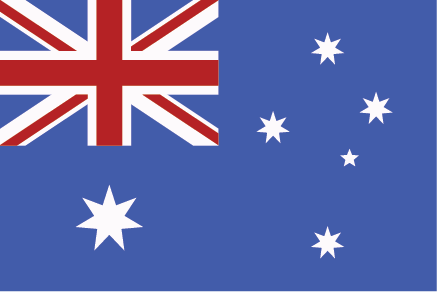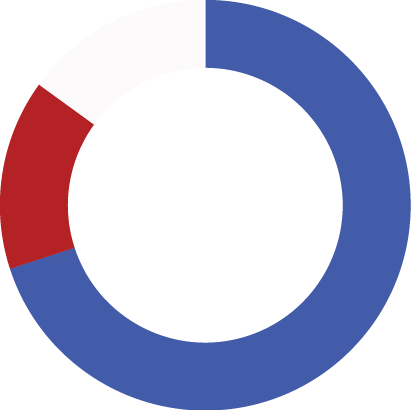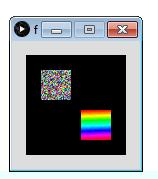#### Howdy, Stranger!

We are about to switch to a new forum software. Until then we have removed the registration on this forum.

# Pixel sorting: Arranging all the pixels from an image in a color wheel. Is it possible?

edited February 2016

I am new to Processing, and I have done quite a lot of searching, but I dont seem to be getting anywhere. My objective is to take all the colours from an image, and arrange them by hue in a colour wheel. I presume the result will represent a pie chart more than an actual spectrum, but that's ok.

I am a designer not a programmer, so integers and arrays are a little alien to me. I have however got code from the GENERATIVE DESIGN book which I thought might work: http://www.generative-gestaltung.de/P_1_1_2_01 for a colour spectrum, http://www.generative-gestaltung.de/P_1_2_2_01 which loads the colour pixels from the image, however does not really arrange them, and uses the mouse x, y coords to tile the colours. It does seem to group similar hues together, which is perfect.

I think what I need is some kind of a cross between these two projects. So firstly, is something like this possible? And if so, am I looking in the right place? Thanks in advance. R

Tagged:

• ``````import generativedesign.*;
import processing.pdf.*;
import java.util.Calendar;

boolean savePDF = false;

int segmentCount = 360;

PImage img;
color[] colors;

String sortMode = GenerativeDesign.HUE;

void setup(){
size(800, 800);
}

void draw(){
if (savePDF) beginRecord(PDF, timestamp()+".pdf");

noStroke();
colorMode(HSB, 360, 100, 100);
background(360);

float angleStep = 360/segmentCount;

// get colors from image
// int i = 0;
//colors = new color[segmentCount*segmentCount];
//for (int gridY=0; gridY<segmentCount; gridY++) {
//for (int gridX=0; gridX<segmentCount; gridX++) {
// int px = (int) (gridX * segmentCount);
// int py = (int) (gridY * segmentCount);
// colors[i] = img.get(px, py);
// i++;
// }
// }

beginShape(TRIANGLE_FAN);
vertex(width/2, height/2);
for (float angle=0; angle<=360; angle+=angleStep){
vertex(vx, vy);
fill(angle, 100, 100);
}
endShape();

if (savePDF) {
savePDF = false;
endRecord();
}
}

// timestamp
String timestamp() {
Calendar now = Calendar.getInstance();
return String.format("%1\$ty%1\$tm%1\$td_%1\$tH%1\$tM%1\$tS", now);
}
``````
• This is the code I am using (not my own), however getting the colors from the image is where I am struggling. For example, using an Australian flag, would hopefully reveal radians within a circle that were roughly 70% blue, 20% red, 10% white. Arranging the pixels by hue within a 360 degree spectrum. Any ideas as to how I could achieve this?

• can you attach the image here?

• ``````/**
* Hue Sorted (v1.0)
* GoToLoop (2016-Feb-28)
*
* forum.Processing.org/two/discussion/15164/
* pixel-sorting-arranging-all-the-pixels-
* from-an-image-in-a-color-wheel-is-it-possible
*/

final int DIAM = 30, GAP = 15;
final int HUES = 90, ATTRIBS = 25;

noLoop();
colorMode(HSB, HUES, ATTRIBS, ATTRIBS);
background(0);

final PImage img = createImage(DIAM, DIAM, ARGB);
final color[] p = img.pixels;

for (int i = 0; i < p.length; p[i++] = (int) random(0xff000000));
img.updatePixels();
set(GAP, GAP, img);

final byte[] hues = new byte[p.length];

for (int i = 0; i < hues.length; hues[i] = (byte) hue(p[i++]));
java.util.Arrays.sort(hues);
printArray(hues);

final IntDict hueDict = new IntDict();

for (byte h : hues)  hueDict.increment(str(h));
println(ENTER, hueDict);

final PImage hueImg = createImage(img.width, img.height, ARGB);
final color[] h = hueImg.pixels;

for (int i = 0; i < h.length; h[i] = color(hues[i++], ATTRIBS, ATTRIBS));
hueImg.updatePixels();
set(width-GAP - hueImg.width, height-GAP - hueImg.height, hueImg);
``````
• Yes sure, thanks for taking a look koogs. GoToLoop thanks for your help, I will have to try that when I get home from work, no processing at work!R

• and a mock up of what you want the output to be like would be good. just a pie chart with red, blue and white in it? (you might get intermediate colours, especially if that's a jpg, but their counts will be small)

gotoloop's (UNDOCUMENTED) code does the hue summing for you, you just need to do the display of the results given an intDict as an input (yuck!)

• Thanks koogs, exactly, just a pie chart, well doughnut to be exact, however I can place an ellipse over the final image to 'mask' out the centre. I know that some intermediate colours will be present, that can't be helped unless I use vectors I suppose, however, the PNG should be ok for now. I hope to use an array of country flags eventually, and when I get this whole thing to work as I want I will upload final results. Thanks again for all your help!• so is that done or just mocked up?

pie charts are easy enough. get the total of all the sums (equal to total pixels in the image) and use arc

https://processing.org/reference/arc_.html

where each arc is the count for the current colour / the total mapped (using map()) from 0 to TWO_PI.

(then a white ellipse in the middle as you say)

• No, just a mock up. But thanks, I will give that a go!

• Ok, excuse the ignorance and yes I am a total newb here. GoToLoop's kind contribution outputs the below:I know I am missing something huge here, I can't seem to load the img, and then shouldn't the output be circular?

• his example was more about summing the hues. the input, top left, is random pixels, the output is the same pixels sorted but, yes, square.

• Ok thanks, I get it. Now I will try decipher :)

• Thanks for everyone's help. I have a long way to go :)

• not really, you have all the bits.

``````for each pixel in image
extract hue
increment total for that hue
end

for each extracted hue
draw segment of pie chart
end
``````

use an IntDict for the incrementing, use the hue as the key

the pie chart is just mapping the ratio of each colour to the range 0 to TWO_PI (or 0 to 360). use arc().

don't worry if you don't understand gotoloop's code - nobody else does either.

• I had some help with this code, however I have two problems to solve: 1. I can't seem to get the values of saturation & brightness. Currently, they get set to 100. I can see why, but I try to create a variable for each of them and fail hopelessly. 2. I will eventually need to load an array of images in, but this is a much more complex issue.

• ```````import generativedesign.*;
import processing.pdf.*;
import java.util.Calendar;

boolean savePDF = false;
PImage img;

void setup(){
size(600, 600);
noStroke();
colorMode(HSB, 360, 100, 100);
background(0, 0, 100);

}

void draw(){

IntDict pixelBuckets = new IntDict();

// Loop through the images pixels
for(int i = 0; i < img.pixels.length;i++) {

// Get the hue as we are keying the dictionary by the hue
float pixelHue = hue(img.pixels[i]);
String key = String.valueOf(pixelHue);

{
// Hue already seen so increment count
int currentCount = pixelBuckets.get(key);
currentCount = currentCount + 1;
pixelBuckets.set(key, currentCount);
}
else
{
// New hue. Start at 1
String newKey = String.valueOf(pixelHue);
pixelBuckets.set(newKey, 1);
}
}

// IntDict has a sort function
pixelBuckets.sortValues();

Integer index = 0;
short[] pieAngles = new short[pixelBuckets.size()];
color[] colors = new color[pixelBuckets.size()];
Integer angleTotal = 0;

for (int i : pixelBuckets.values()) {

String key =  pixelBuckets.keyArray()[index];
float hue = Float.parseFloat(key);
color theColor = color(Math.round(hue), 100, 75);

println("Color: " + theColor + " occurs " + i + " times");

float percentage =  (i * 100.0f) / img.pixels.length;
//short percentageAsShort = (short) Math.round(percentage);

// println("Percentage is:" + percentage + "%");
// println("Percentage (short) is:" + percentageAsShort + "%");

//println(" | hsb: " + int(hue(c)) + "," + int(saturation(c)) + "," + int(brightness(c)));

short angle = (short)Math.round((360 * (percentage / 100)));
angleTotal = angleTotal + angle;

if (index == pixelBuckets.size() - 1)
{
// Last peice of pie to be calculated. Ensure ou total sum of angles adds up to 360
// to avoid gaps. The reason it does not always round up is likely to be rounding issues

Integer diff = 360 - angleTotal;
pieAngles[index] = (short)(angle + diff);
}
else
{
pieAngles[index] = angle;
}

if (angle >= 2)
{
colors[index] = theColor;
}
else
{
// Anything less than a certain angle ignore. Set to -1 and later those colors to be the most common color
colors[index] = -1;
}

index++;
}

//println("HashMap Size " + pixelBuckets.size());

for (int colorIndex = 0; colorIndex < colors.length; colorIndex++)
{
if (colors[colorIndex] == -1)
{
colors[colorIndex] = colors[colors.length - 1];
}
}

drawPieChart(pieAngles, colors, height*3 >> 2);
fill(0, 0, 100);
ellipse(width>>1, height>>1, width/2, height/2);

noLoop();
}

void drawPieChart(short[] angles, color[] colors, int diam) {
final int rad = diam>>1, cw = width>>1, ch = height>>1;
int idx = angles.length;
float angSum = 0;

while (idx-- != 0) {